WHCSRL 技术网

# 数据结构KMP算法详解（超详细）

## 一、什么是KMP算法？

KMP算法是一种改进的字符串匹配算法，由D.E.Knuth，J.H.Morris和V.R.Pratt提出的，因此人们称它为克努特—莫里斯—普拉特操作（简称KMP算法）。KMP算法是在 BF 算法基础上改进得到的算法。学习 BF 算法我们知道，该算法的实现过程就是 “傻瓜式” 地用模式串（假定为子串的串）与主串中的字符一一匹配，匹配不成功则返回到上一次与主串匹配的下一位字符进行匹配，算法执行效率不高。

## 二、KMP算法的解决题型

KMP算法是在数据结构中两个字符串相互匹配衍生出来的算法。KMP算法的作用是在一个已知字符串中查找子串的位置,也叫做串的模式匹配。例如，对主串 A（“ABCABCE”）和模式串 B（“ABCE”）进行模式匹配，如果人为去判断，仅需匹配两次。虽然在以上字符较少的串中人为匹配很容易，但是让计算机来匹配就相对慢一些，但是当字符串中的字符非常多的时候，就不可能人为去匹配。所以打铁还需自身硬，我们把这种枯燥的事以一定的算法交给计算机处理。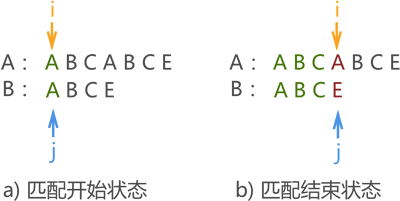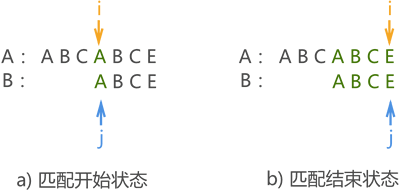## 三、模式串移动距离的判断（next数组）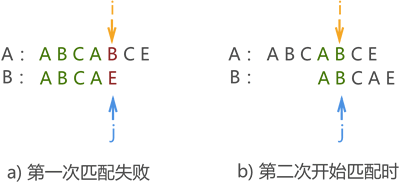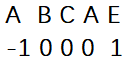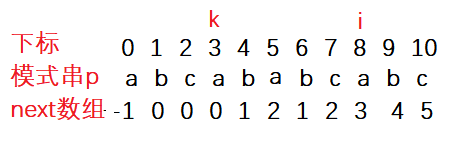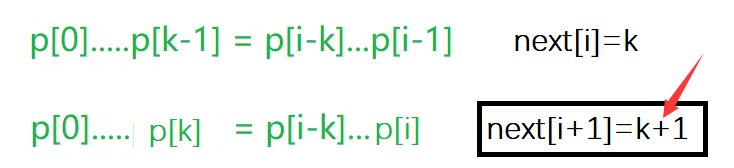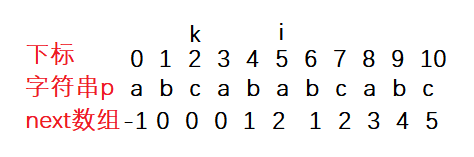1. next数组的值每次只能加1，不能跳着加，否则一定是错的。
2. 当确定将要确定某个字符对应的next的值并且与它的下标为k的字符不相同时，将要确定某个字符的next值不一定要从0开始。
3. 用编程来实现next数组需要注意的是我们不知道第i的next值为多少，但是我们知道只要有p[i]=p[k]，则必定有next[i+1]=k。因此我们要从前往后推。只在next数组的下标为0的值放入-1，其他的next值都进行“知前往后”推导。

``````#include <stdio.h>
#include <string.h>
#include <assert.h>
#include <stdlib.h>
void GetNext(char* arr2, int* next)//arr2为字串
{
int i = 0;
int k = -1;
int len2 = strlen(arr2);
next = -1;
while (i < len2)
{
if (k == -1 || arr2[i] == arr2[k])//k==-1时有两种情况：第一种是一开始k就为-1，第二种是k=-1是不能再回溯。
{
k++;//若相等则k一定只加1
i++;//子串往后移一位里放next的值
next[i] = k;//放入next的值
}
else
{
k = next[k];//字符不同则回溯
}
}
}
int KMP(char* arr1, char* arr2) //arr1为主串，arr2为字串
{
assert(arr1 && arr2); //保证传入的指针不是空指针
int len1 = strlen(arr1);
int len2 = strlen(arr2);
if (len2<0 || len2>len1)
{
return -1;
}
if (len1 == 0 || len2 == 0)   //两种不可能的情况
{
return -1;
}
int* next = (int*)malloc(sizeof(int) * len2);//为next数组动态开辟空间
GetNext(arr2, next);
int i = 0;
int j = 0;
while (j == -1 || i < len1 && j < len2)
{
if (arr1[i] == arr2[j])//比较相等均后移一位
{
i++;
j++;
}
else
{
j = next[j];//不相等则回溯子串，主串不动
}
}
if (j >= len2)
{
return i - j;//返回主串与字串开始匹配到成功的开始位置
}
return -1;//子串与主串没有可匹配的字符串
}
int main()
{
char arr1[] = "abababcabc";
char arr2[] = "abcabc";
printf("%%%%d", KMP(arr1, arr2));
return 0;
}
```123456789101112131415161718192021222324252627282930313233343536373839404142434445464748495051525354555657585960616263646566```

## 四、KMP算法的具体实现

• 第一次匹配如图八所示，匹配结果失败，指针 j 移动至 next[j] 的位置：
图八：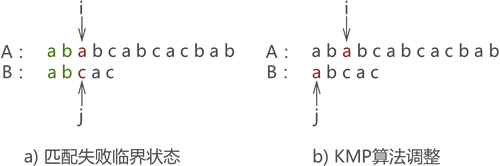• 第二次匹配如图九所示，匹配结果失败，依旧执行 j=next[j] 操作：
图九：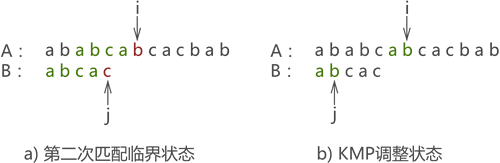• 第三次匹配成功，如图十所示：
图十：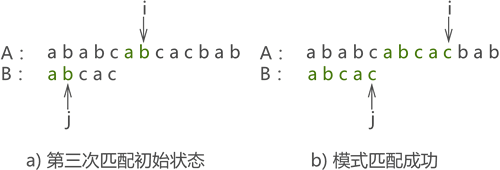很明显，使用 KMP 算法只需匹配 3 次，而同样的问题使用 BF 算法则需匹配 6 次才能完成。

## 五、KMP算法的时间复杂度

KMP算法中多了一个求数组的过程，多消耗了一点点空间。我们设主串s长度为n,子串t的长度为m。求next数组时时间复杂度为O(m)，因后面匹配中主串不回溯，比较次数可记为n，所以KMP算法的总时间复杂度为O(m+n)，空间复杂度记为O(m)，只为next数组开辟了m个int字节大小的空间。相比于朴素的模式匹配时间复杂度O(m*n)，KMP算法提速是非常大的。

## 六、next数组的改进–nextval数组及具体代码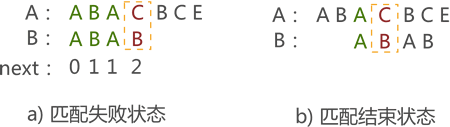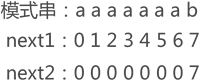next数组改进后的代码：

``````#include <stdio.h>
#include <string.h>
#include <assert.h>
#include <stdlib.h>
void GetNext(char* arr2, int* next)
{
int i = 0;
int k = -1;
int len2 = strlen(arr2);
next = -1;
while (i < len2)
{
if (k == -1 || arr2[i] == arr2[k])
{
k++;
i++;
if (arr2[i] == arr2[k])//进入第一个if语句后向后一位的字符该arr2[i]与arr2[k]仍然相等则直接一次性回溯到位
{
next[i] = next[k];
}
else     //arr2[i] ！= arr2[k]
{
next[i] = k; //不用多次回溯，跟next数组一样
}
}
else
{
k = next[k];
}
}
}
int KMP(char* arr1, char* arr2)
{
assert(arr1 && arr2);
int len1 = strlen(arr1);
int len2 = strlen(arr2);
if (len2<0 || len2>len1)
{
return -1;
}
if (len1 == 0 || len2 == 0)
{
return -1;
}
int* next = (int*)malloc(sizeof(int) * len2);
GetNext(arr2, next);
int i = 0;
int j = 0;
while (j == -1 || i < len1 && j < len2)
{
if (arr1[i] == arr2[j])
{
i++;
j++;
}
else
{
j = next[j];
}
}
if (j >= len2)
{
return i - j;
}
return -1;
}
int main()
{
char arr1[] = "abababcabc";
char arr2[] = "abcabc";
printf("%%%%d", KMP(arr1, arr2));
return 0;
}
```12345678910111213141516171819202122232425262728293031323334353637383940414243444546474849505152535455565758596061626364656667686970717273```